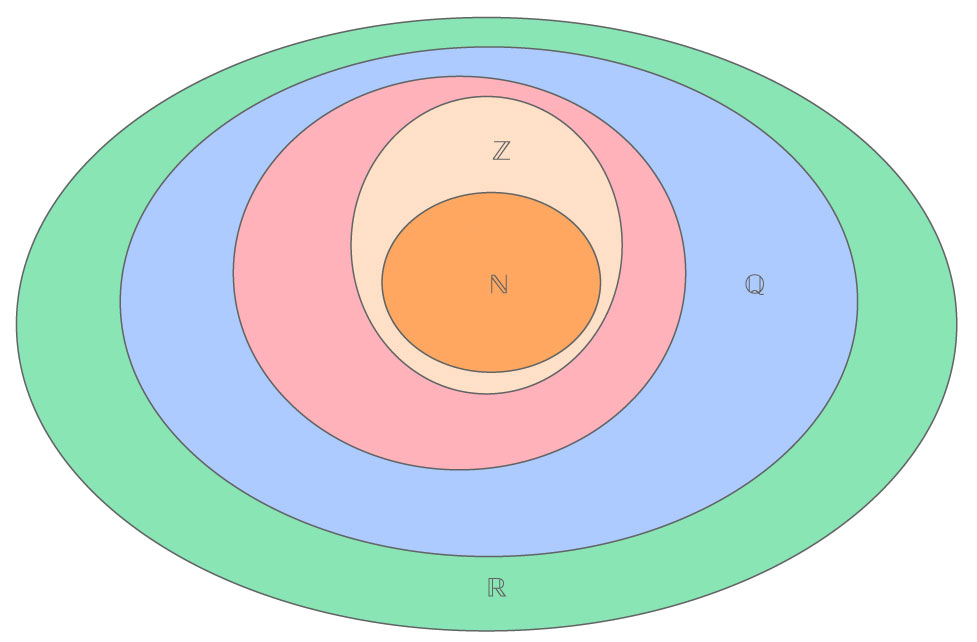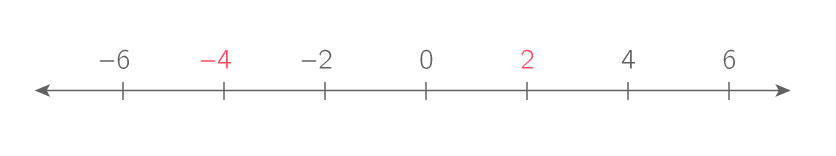Fundamentals of numbers Lesson

I

Write basic expressions and equations

Mathematical expression

A mathematical expression is an expression involving mathematical symbols such as numbers, variables, and mathematical operators.

$$\displaystyle{\dfrac{2}{3}x+17e^x}$$

$$\displaystyle{\sin\left(\dfrac{\pi}{2}\right)-1}$$

Equation

An equation is a statement of equality of two mathematical expressions.

We can have the following equation :

$$\displaystyle{2x+2=4}$$

The goal is to find the value of x that makes the statement " $$\displaystyle{2x+2=4}$$ " true.

$$\displaystyle{\sin\left(\dfrac{\pi}{2}\right)-1=0}$$

When it comes to solving problems, it is useful to be able to translate between written words and mathematical expressions and equations.

For example, the sentence "the sum of 18 and y is 20" can be written as :

$$\displaystyle{18+y=20}$$

Consider the following problem: Bill is currently twelve years older than Janet, and in two years Bill will be twice the age of Janet. How old is Janet?

To solve such a problem we will need to come up with mathematical equations which will need to be solved. If we let $$\displaystyle{B}$$ denote the current age of Bill and $$\displaystyle{J}$$ denote the current age of Janet then the phrase "Bill is currently twelve years older than Janet" is mathematically expressed as

$$\displaystyle{B=J+12}$$

and the phrase " in two years Bill will be twice the age of Janet" is mathematically expressed as

$$\displaystyle{B+2=2 J}$$.

We can now use the method of substitution to find Janet's age, but that will be discussed in a later section.

II

Number Classification

A

The real numbers system and its internal classification

Number

A number is a mathematical object used to count and measure.

$$\displaystyle{0, 1, 2, 3, -3, -2, -1, \pi, 3.11,}$$ $$\displaystyle{\dfrac{7}{2}, \sqrt{-1}}$$, are all examples of numbers.

Real number

A real number is any quantity, which can be either positive or negative, that can be used to represent the distance along a line. The set of all real numbers is denoted $$\displaystyle{\mathbb{R}}$$.

The numbers $$\displaystyle{0,1-2,\pi, 3.11 \text{ or } \dfrac{7}{2}}$$ are all real numbers.

The number $$\displaystyle{\sqrt{-1}}$$ is not a real number.

Integer

An integer is a whole number which can be either positive, negative, or $$\displaystyle{0}$$. The set of all integers is denoted $$\displaystyle{\mathbb{Z}}$$.

The numbers $$\displaystyle{−12, 4, −756}$$ and $$\displaystyle{3542}$$ are all integer numbers.

The numbers $$\displaystyle{−14.35}$$ and $$\displaystyle{46.5}$$ are not integer numbers.

Natural Number

A natural number is an integer which is nonnegative. The set of all natural numbers is denoted $$\displaystyle{\mathbb{N}}$$.

0, 4, 9, 136 and 9078 are all natural numbers.

−3 and 1.83 are not natural numbers.

Rational Number

A rational number is any real number which can be expressed as the ratio $$\displaystyle{\dfrac{a}{b}}$$ of two integers $$\displaystyle{a,b}$$ with $$\displaystyle{b\neq0}$$. The set of all rational numbers is denoted $$\displaystyle{\mathbb{Q}}$$.

$$\displaystyle{\dfrac{3}{12}}$$ is a rational number.

The numbers $$\displaystyle{\sqrt{2}}$$ and $$\displaystyle{\pi}$$ are not rational numbers and are referred to as irrational numbers.

Sometimes, the decimal expression of a real number involves a repeating decimal pattern.

The decimal expression of the fraction $$\displaystyle{\dfrac{1}{3}}$$ is $$\displaystyle{0.333\ 333\ 333\ldots}$$ and the decimal expression will continue forever.

To express that the pattern of 3 repeats forever in the decimal expression we write $$\displaystyle{0.3\overline{3}}$$.

The decimal expression of the fraction $$\displaystyle{\dfrac{13}{99}}$$ is:

$$\displaystyle{0.\overline{13}=0.13\ 131\ 313\ldots}$$

The different sets of numbers are included in each other, as shown on the image below:B

Introduction to the complex number system

The number i

The symbol $$\displaystyle{i}$$ is used to denote $$\displaystyle{\sqrt{-1}}$$. That is $$\displaystyle{i}$$ is a number such that:

$$\displaystyle{i^2=−1}$$

The square of any real number cannot be negative. Therefore the number $$\displaystyle{i}$$ is not a real number.

Complex Number

Let a and b be two real numbers. A complex number is any number of the form:

$$\displaystyle{z=a+bi}$$

$$\displaystyle{z_1=\sqrt{2}-3i}$$ and $$\displaystyle{z_2=2+i}$$ are examples of complex numbers.

The complex number system is an extension of the real number system which allows us to have numbers whose squares can be negative.

Every real number is a complex number. The set of real numbers is included in the set of complex numbers.

2 is a real number. It can also be written as $$\displaystyle{2+0.i}$$, therefore it is a complex number.

If a and b are real numbers, then the complex number $$\displaystyle{a+bi}$$ is real if and only if $$\displaystyle{b=0}$$.

III

Basic operations with real numbers

A

Compare and order real numbers

Compare real numbers

Comparing two real numbers means assessing which one is greater than the other. Given two real numbers a and b :

• If a is greater than b, we write $$\displaystyle{a\gt b}$$.
• If b is greater than a, we write $$\displaystyle{a \lt b}$$.

The number 7.89 is greater than 2.4. Therefore :

$$\displaystyle{2.4 \lt 7.89}$$

Given two real numbers a and b :

• If a is greater than b or equal to b, we write $$\displaystyle{a\geqslant b}$$
• If b is greater than a or equal to a, we write $$\displaystyle{a \leqslant b}$$

The number $$\displaystyle{7}$$ equals the number $$\displaystyle{7}$$. Therefore:

$$\displaystyle{7\leq 7}$$

And:

$$\displaystyle{7\geq 7}$$

If $$\displaystyle{a\mbox{ and }b}$$ real numbers, then $$\displaystyle{a\leq b}$$ if and only if $$\displaystyle{a}$$ is further to the left than $$\displaystyle{b}$$ on the real number line.On the number line, $$\displaystyle{−4}$$ is further to the left than $$\displaystyle{2}$$ and so $$\displaystyle{−4\leq 2}$$.

Order real numbers

To order a collection of real numbers means to compare all the numbers in the collection.

Given 3.41, $$\displaystyle{\pi}$$, −54, 8.903 and 0 we can write :

$$\displaystyle{−54\lt 0\lt 8.903\lt \pi\lt 3.41}$$

B

Addition, substraction, multiplication and division of real numbers

The operations of addition, subtraction, multiplication, and division take two real numbers and produce a new real number.

If $$\displaystyle{a\mbox{ and }b}$$ are real numbers and $$\displaystyle{b\geq 0}$$, then the number $$\displaystyle{a+b}$$ is the number obtained by increasing the size of $$\displaystyle{a}$$ by the size of $$\displaystyle{b}$$.

The sum of $$\displaystyle{3}$$ and $$\displaystyle{2}$$ is:

$$\displaystyle{3+2=5}$$

Subtraction

If $$\displaystyle{a\mbox{ and }b}$$ are real numbers with $$\displaystyle{b\geq 0}$$ then the number $$\displaystyle{a-b}$$ is the number obtained by decreasing the size of $$\displaystyle{a}$$ by the size of $$\displaystyle{b}$$.

Subtracting $$\displaystyle{2}$$ from $$\displaystyle{3}$$ produces:

$$\displaystyle{3−2=1}$$

If $$\displaystyle{a\mbox{ and }b}$$ are any real numbers, then we can still interpret $$\displaystyle{a+b\mbox{ and }a-b}$$. If $$\displaystyle{b}$$ is negative then $$\displaystyle{-b}$$ is positive, $$\displaystyle{a+b=a- \left(-b\right)}$$, and $$\displaystyle{a-b=a+\left(-b\right)}$$. Therefore we can interpret $$\displaystyle{a+b}$$ as a subtraction and $$\displaystyle{a-b}$$ as an addition.

The sum of 14 and −5 is:

$$\displaystyle{14+\left(-5\right)=14-5=9}$$

Substracting −5 from 4 produces:

$$\displaystyle{4-\left(-5\right)=4+5=9}$$

Multiplication

If $$\displaystyle{a\mbox{ and }b}$$ are real numbers with $$\displaystyle{b\geq 0}$$ then the number $$\displaystyle{ab}$$ is the number obtained by scaling the number $$\displaystyle{a}$$ by the number $$\displaystyle{b}$$.

Multiplying $$\displaystyle{3}$$ and $$\displaystyle{4}$$ produces:

$$\displaystyle{3\cdot 4=12}$$

If $$\displaystyle{a\mbox{ and }b}$$ are real numbers and $$\displaystyle{b \leq 0}$$ then $$\displaystyle{-b\geq 0}$$ and we let $$\displaystyle{a\cdot b= - a\cdot \left(-b\right)}$$.

If $$\displaystyle{a}$$ is any real number then:

$$\displaystyle{a\cdot 0=0}$$

The number $$\displaystyle{17 321}$$ multiplied by $$\displaystyle{0}$$ produces:

$$\displaystyle{17 321\cdot 0=0}$$

Division

If a and b are real numbers (with $$\displaystyle{b\neq0}$$ ) then $$\displaystyle{\dfrac{a}{b}}$$ is the real number such that $$\displaystyle{b\cdot \dfrac{a}{b}=a}$$.

The number $$\displaystyle{34}$$ divided by $$\displaystyle{17}$$ produces:

$$\displaystyle{\dfrac{34}{17}=2}$$

The reason $$\displaystyle{\dfrac{34}{17}=2}$$ is because $$\displaystyle{17\cdot 2=34}$$.

If $$\displaystyle{a}$$ is a real number, then $$\displaystyle{\dfrac{a}{0}}$$ is undefined.